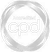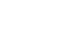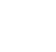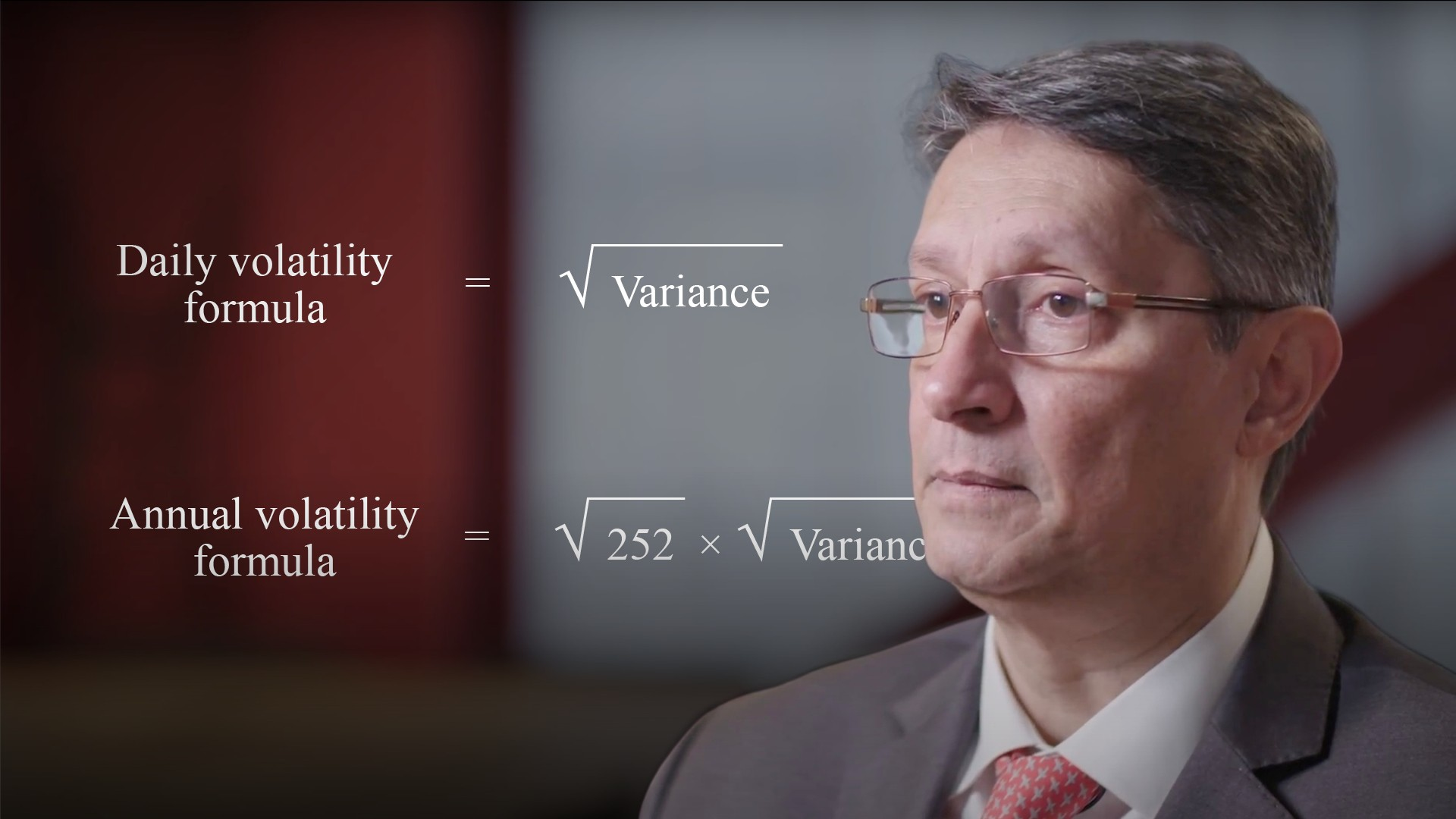# Measuring Volatility### Abdulla Javeri

Volatility can be measured for any period. In this video, Abdulla explains the two ways to convert from one period to another.

Financial MathsVolatility can be measured for any period. In this video, Abdulla explains the two ways to convert from one period to another.

Finance Unlocked is the video learning platform built for finance professionals.### Measuring Volatility

4 mins 34 secs

Overview

Volatility can be measured in two ways – the direct method and the variance method. Both are explained and calculated.

Key learning objectives:

• Calculate volatility using the variance method and the direct method

## Book a demo to access

Summary

#### How do we measure volatility?

Volatility can be measured for any period that we want. To convert into an annualised figure or for any other period takes a bit of simple manipulation. For example if we know that the annual volatility is 15%, we can work out the equivalent quarterly or daily volatility. Or if we have a daily volatility of 0.20%, we can work out what that means in terms of an annual figure and so on. There are two ways to convert from one period to another. Directly, which involves using square roots because volatility is a function of the square root of time, or by applying the variance method. That’s possibly more intuitive, but involves more steps.

#### How do we calculate volatility using the direct method?

To convert directly we take the volatility figure for the period we have and multiply or divide by the relevant ratio to get the figure for the period we want. The ratio is the square root of the number of days in the longer period divided by the number of days in the shorter period. Multiply if going from a shorter to a longer period and divide to go from a longer period to a shorter one.

#### How do we calculate volatility using the variance method?

Moving on to the variance method. Variance is linear with respect to time. Take the measured volatility, and square it. Multiply it by the ratio of long period days to short period days if going from short to long, or divide, if moving from long to short. Finally, take the square root of the result.

## Book a demo to access

Expert### Abdulla Javeri

Abdulla’s career in the financial markets started in 1990 when he entered the trading floor of the London International Financial Futures Exchange, LIFFE, and qualified as a pit trader in equity and equity index options. In 1996, Abdulla became a trainer for regulatory qualifications and then for non-exam courses, primarily covering all major financial products.03:18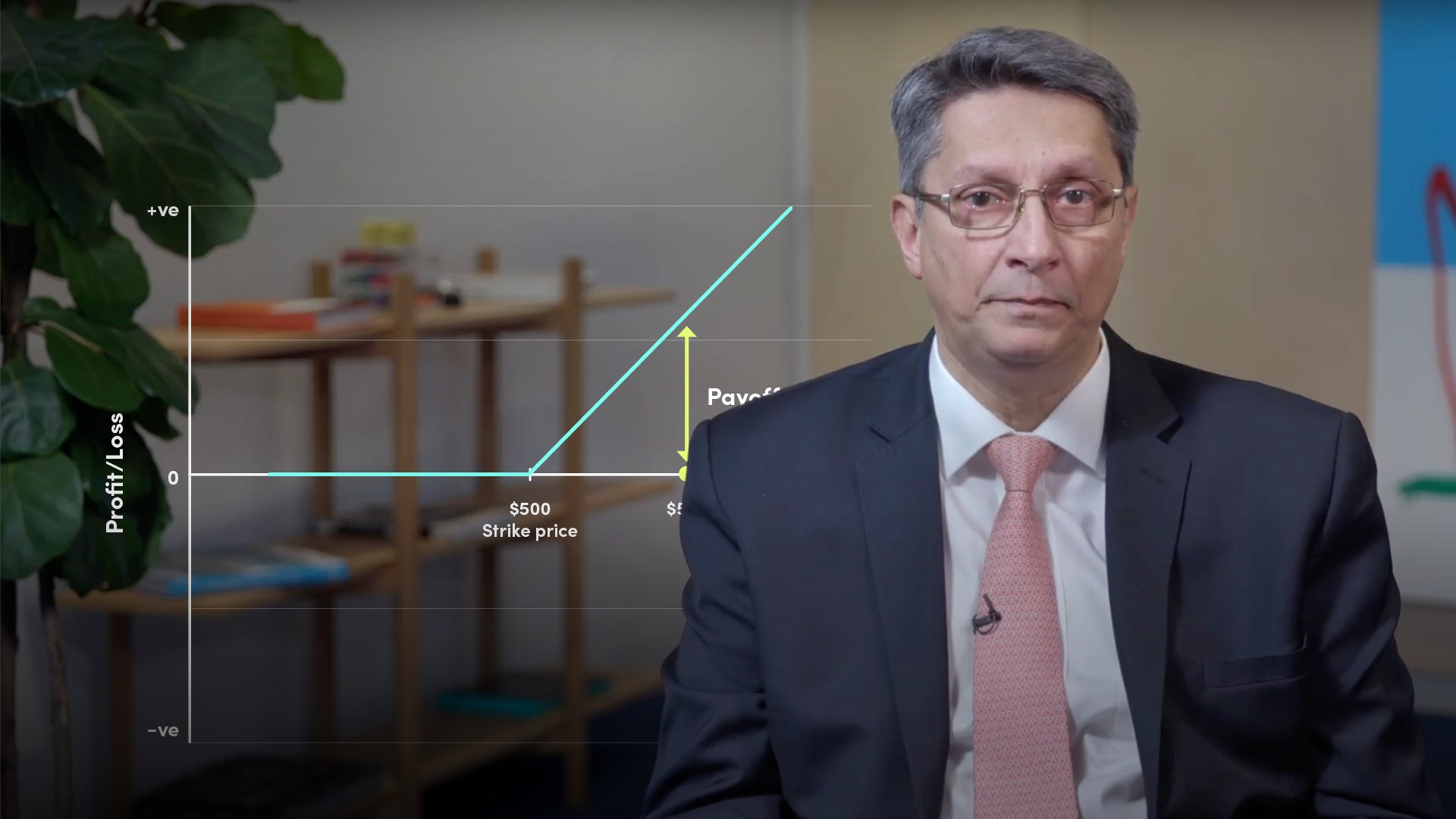05:1205:39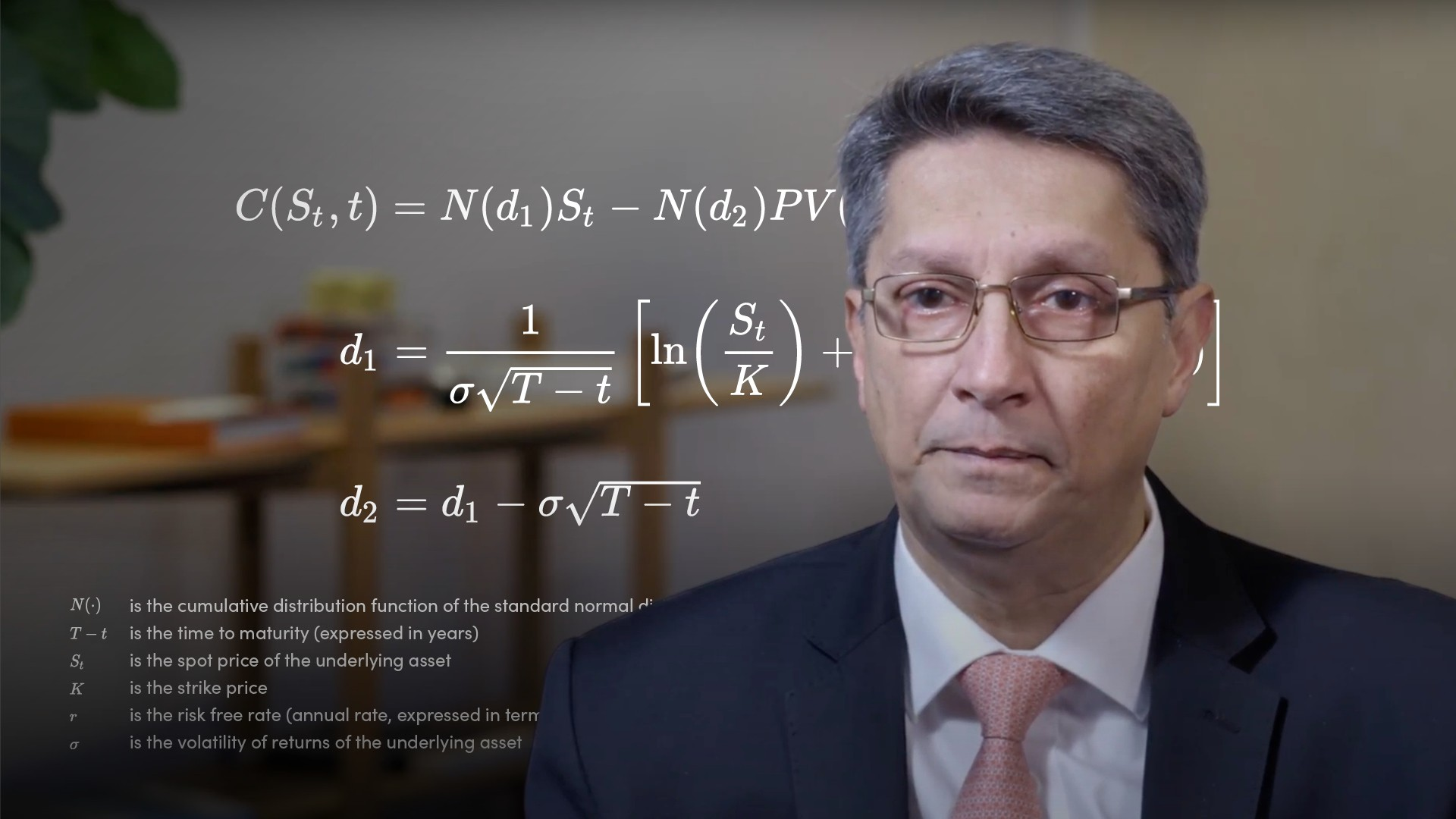05:0804:52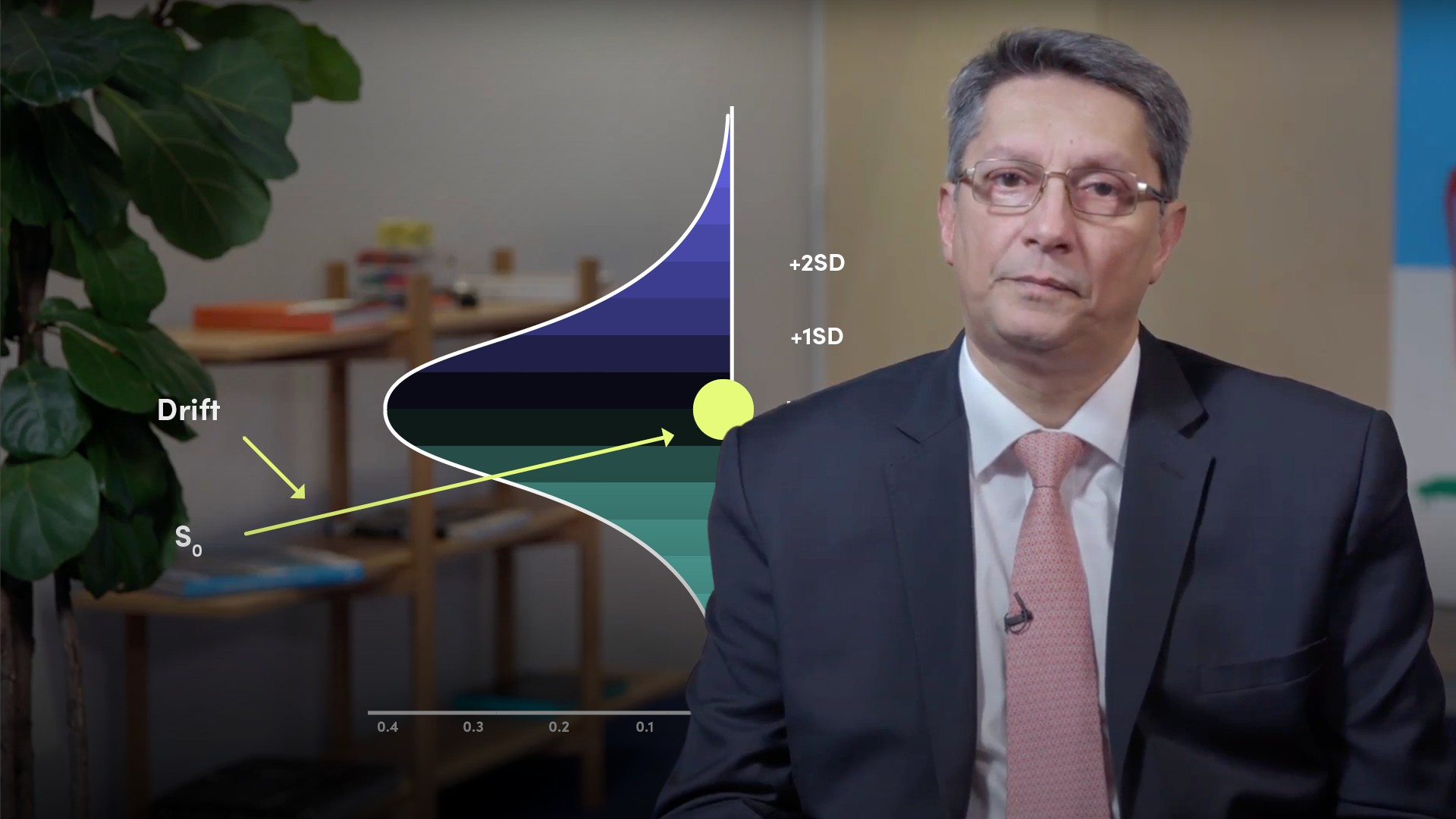05:0904:1305:3804:0804:2205:0203:26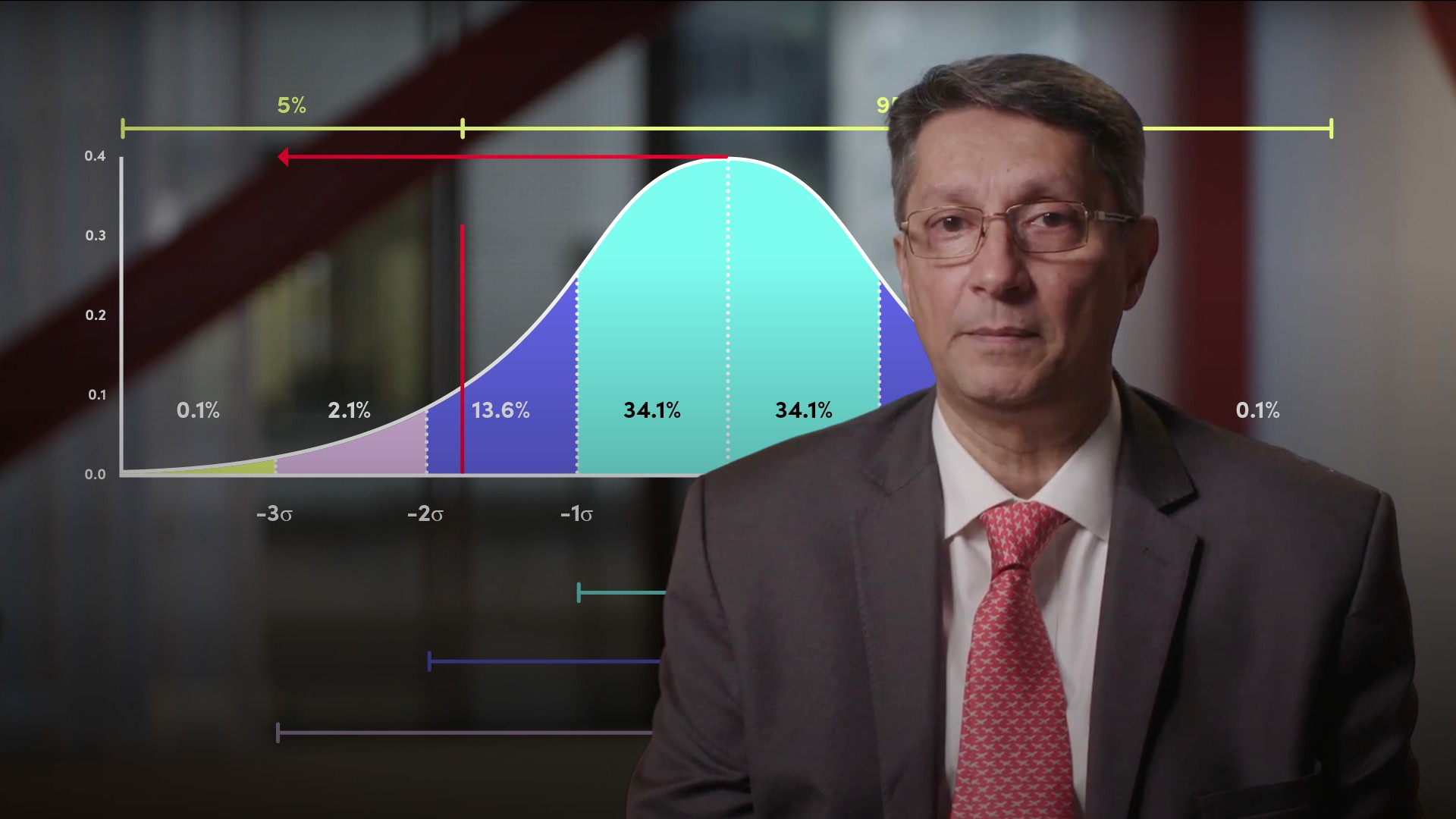03:5804:55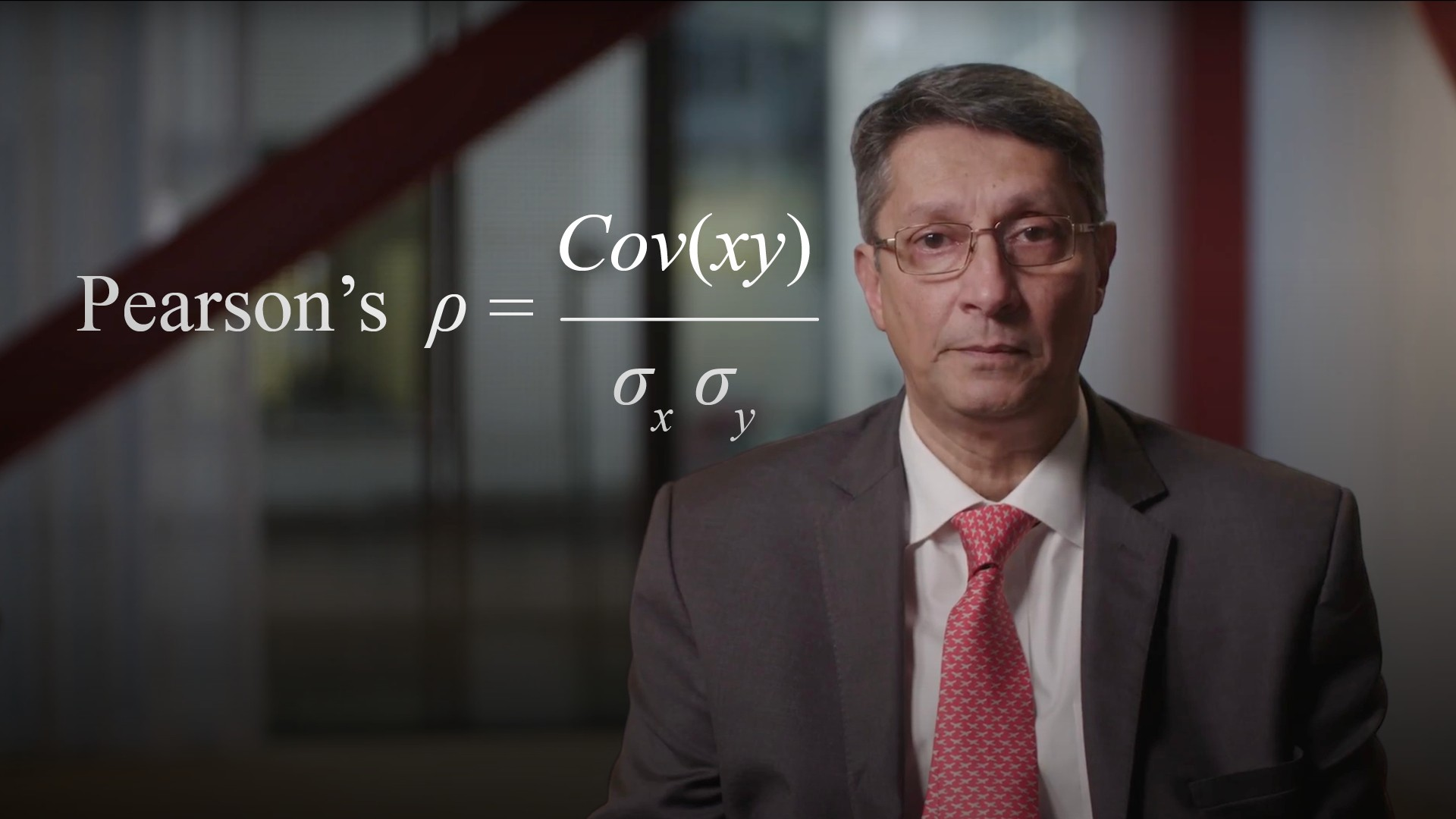04:5604:4005:42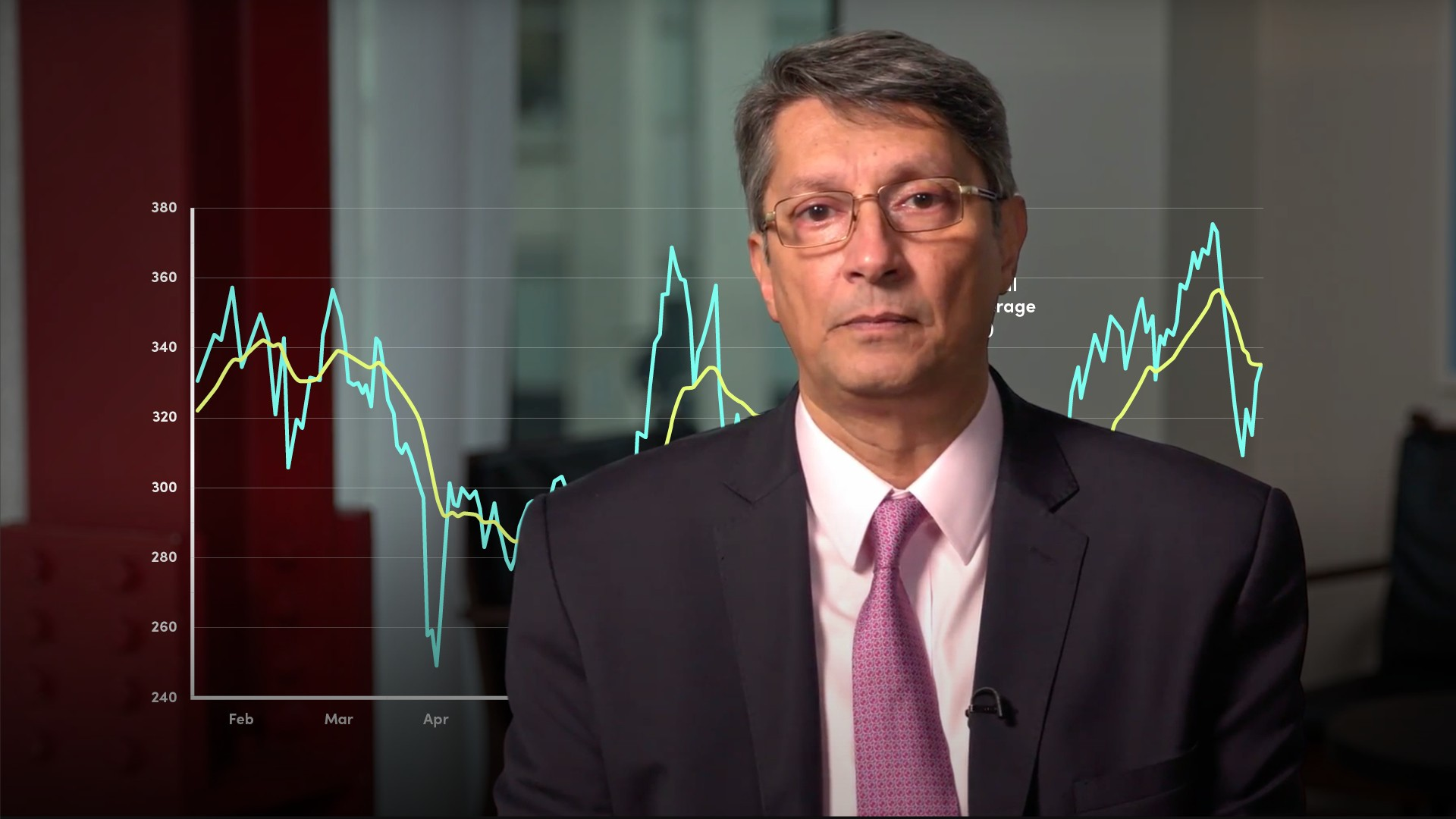05:1104:4903:5806:4403:49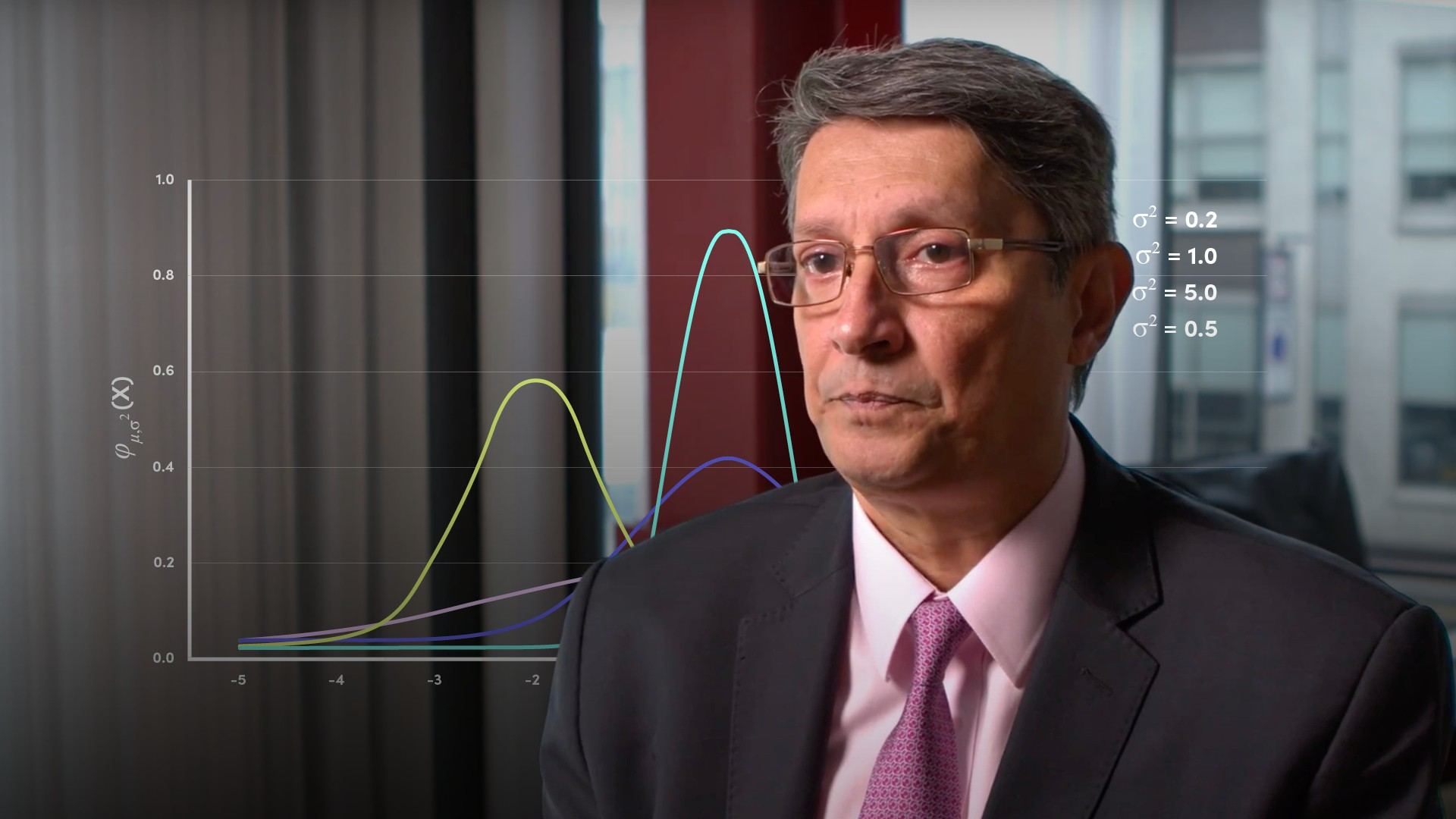04:57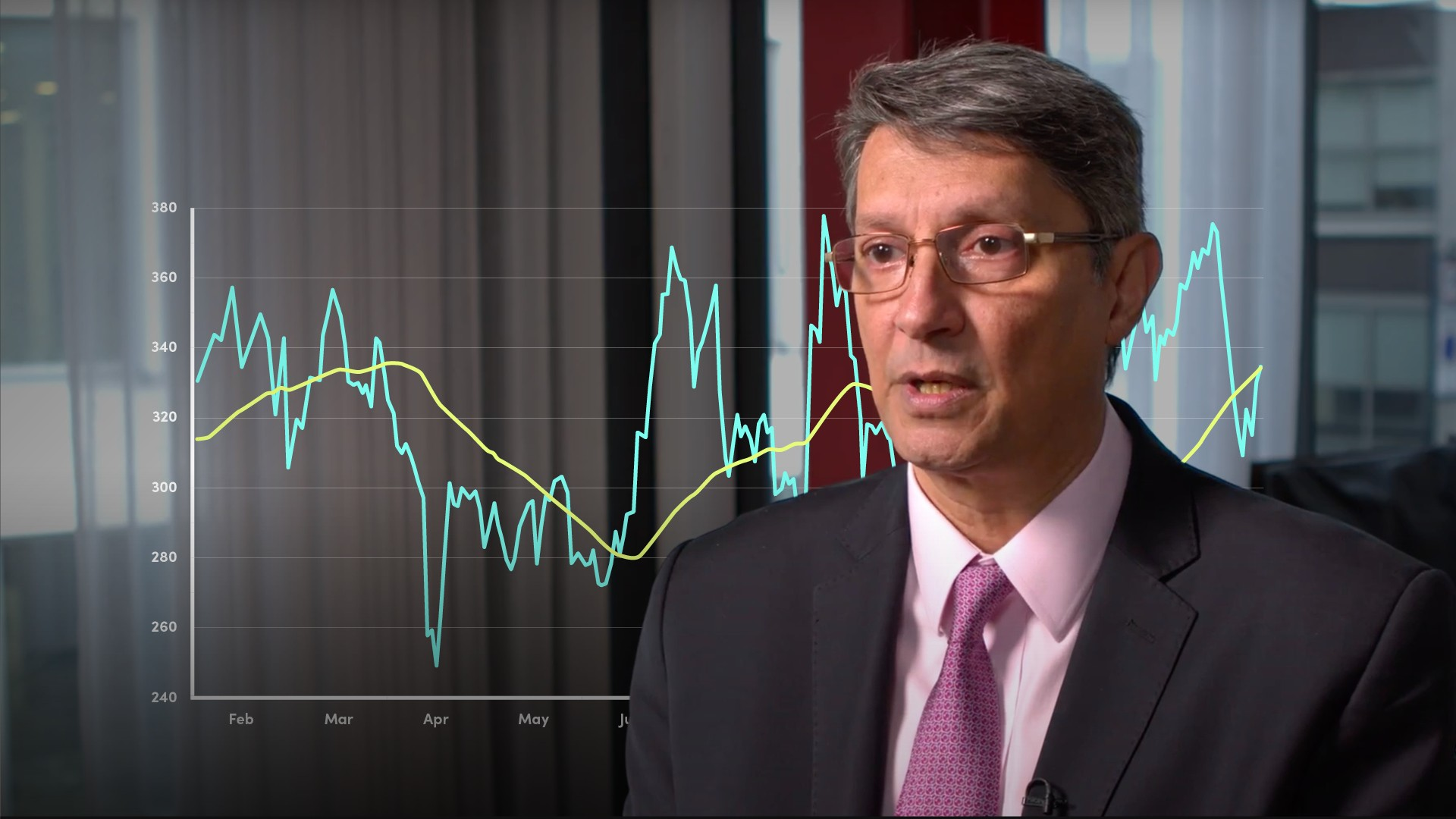04:0004:11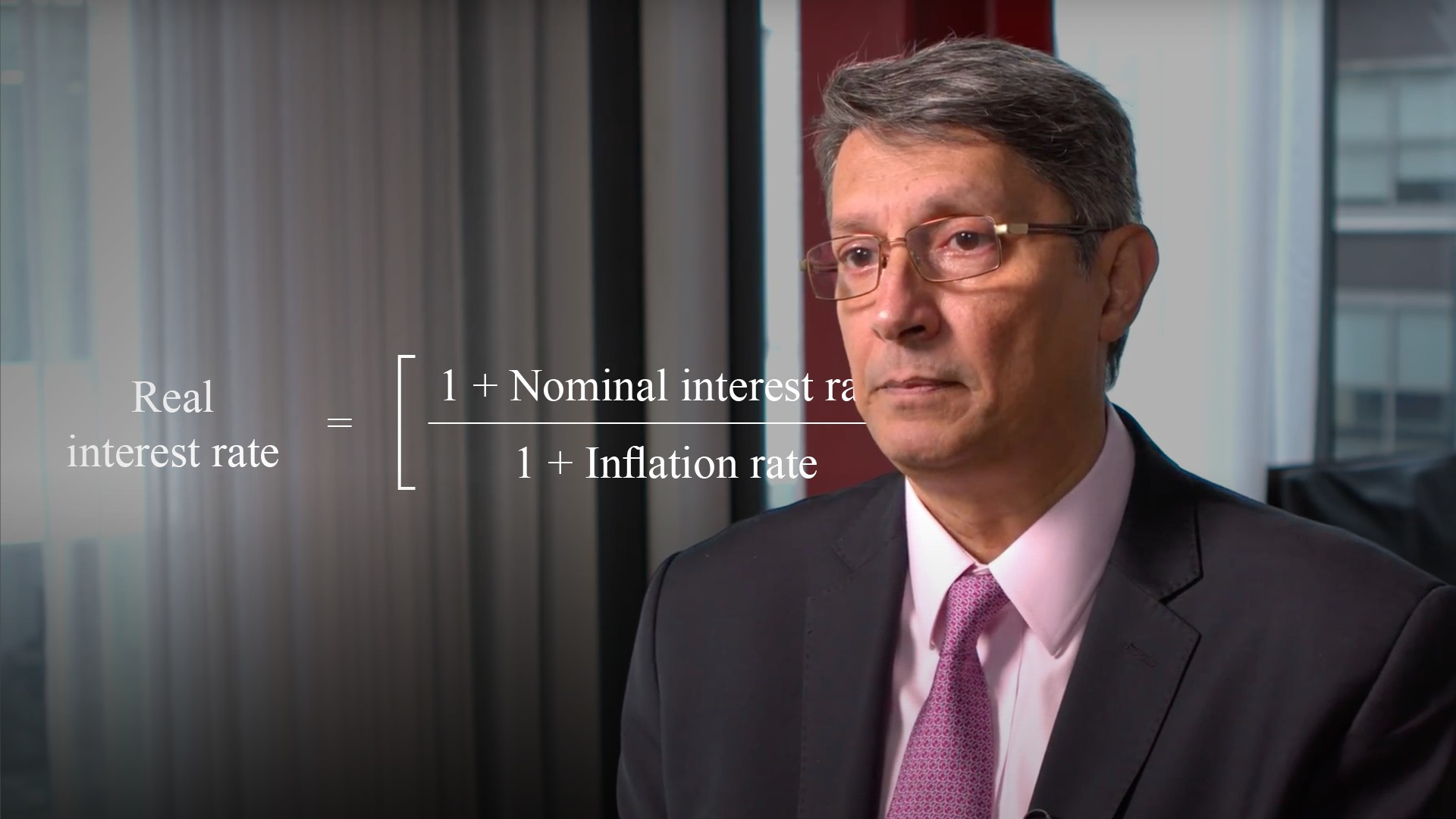04:4205:0304:3704:36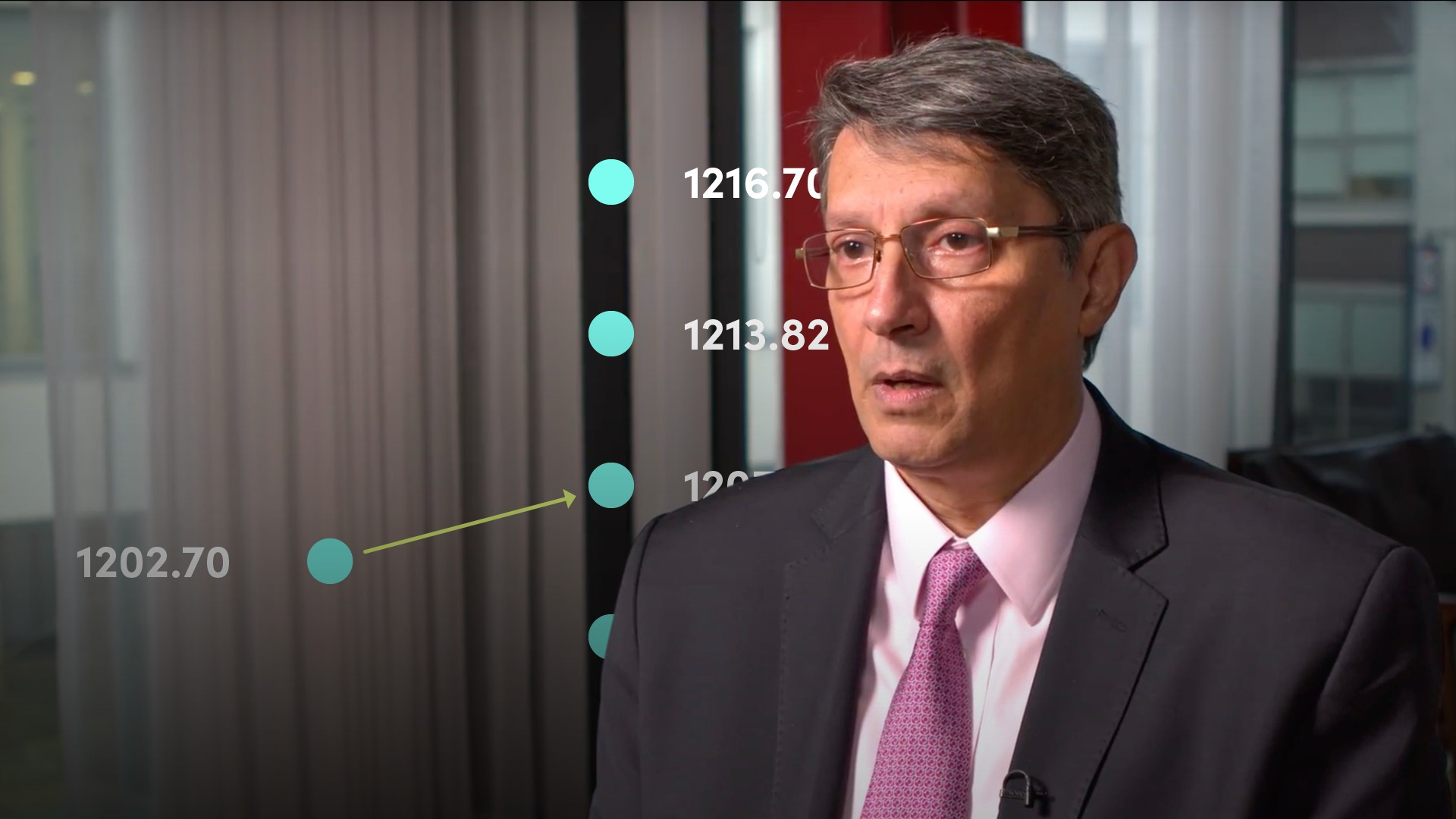06:3505:00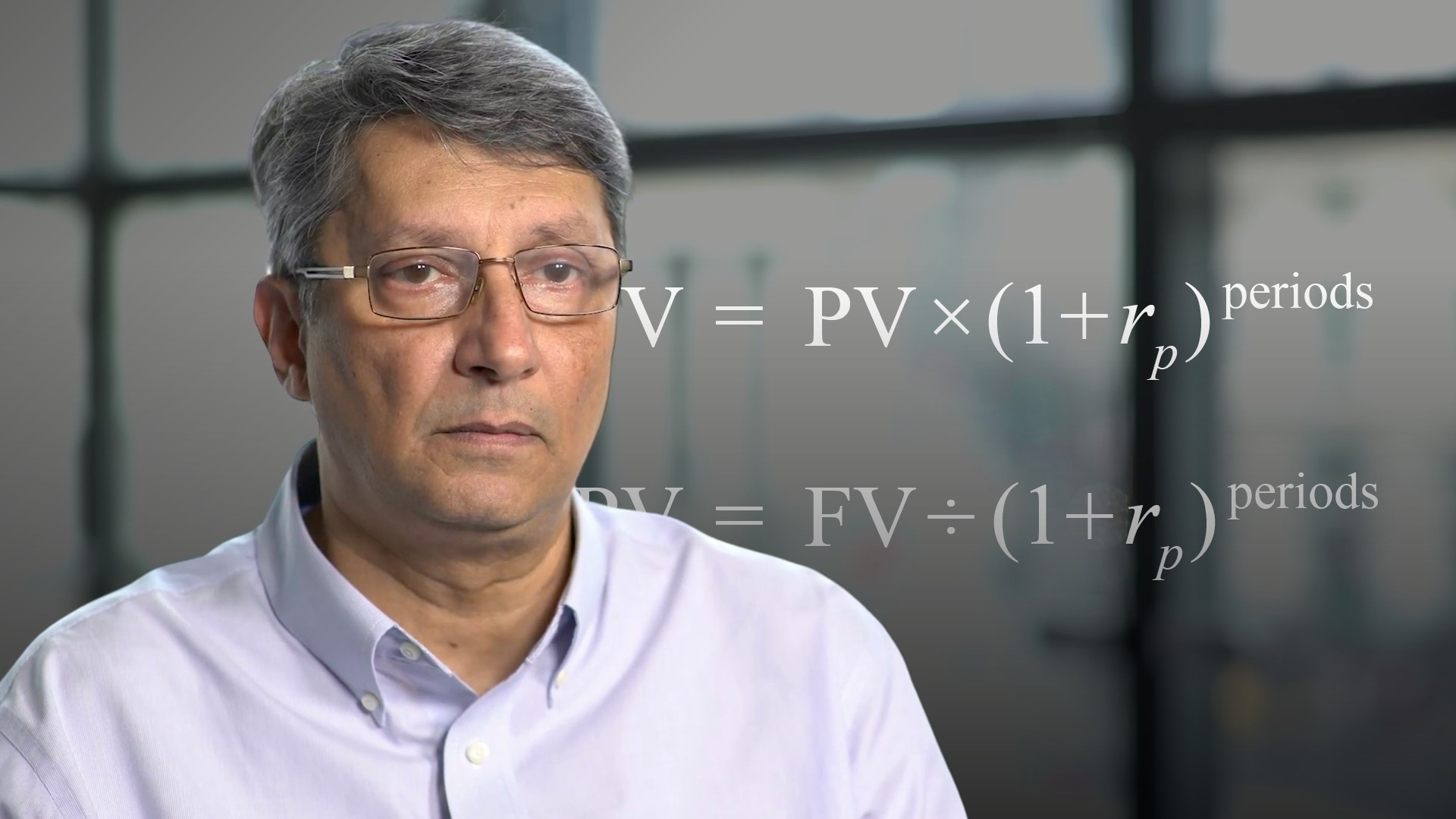03:1006:0805:2805:2304:04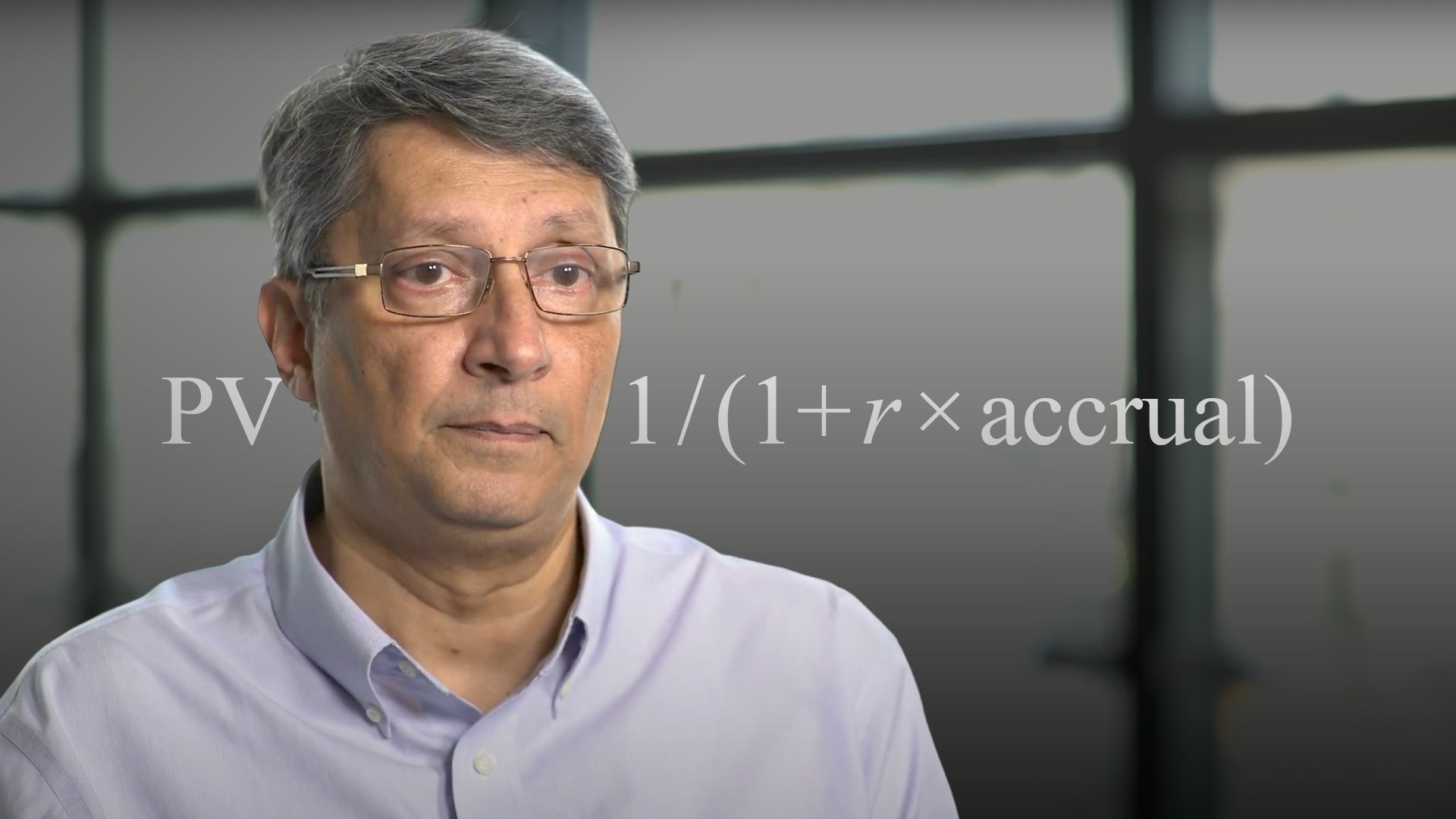03:46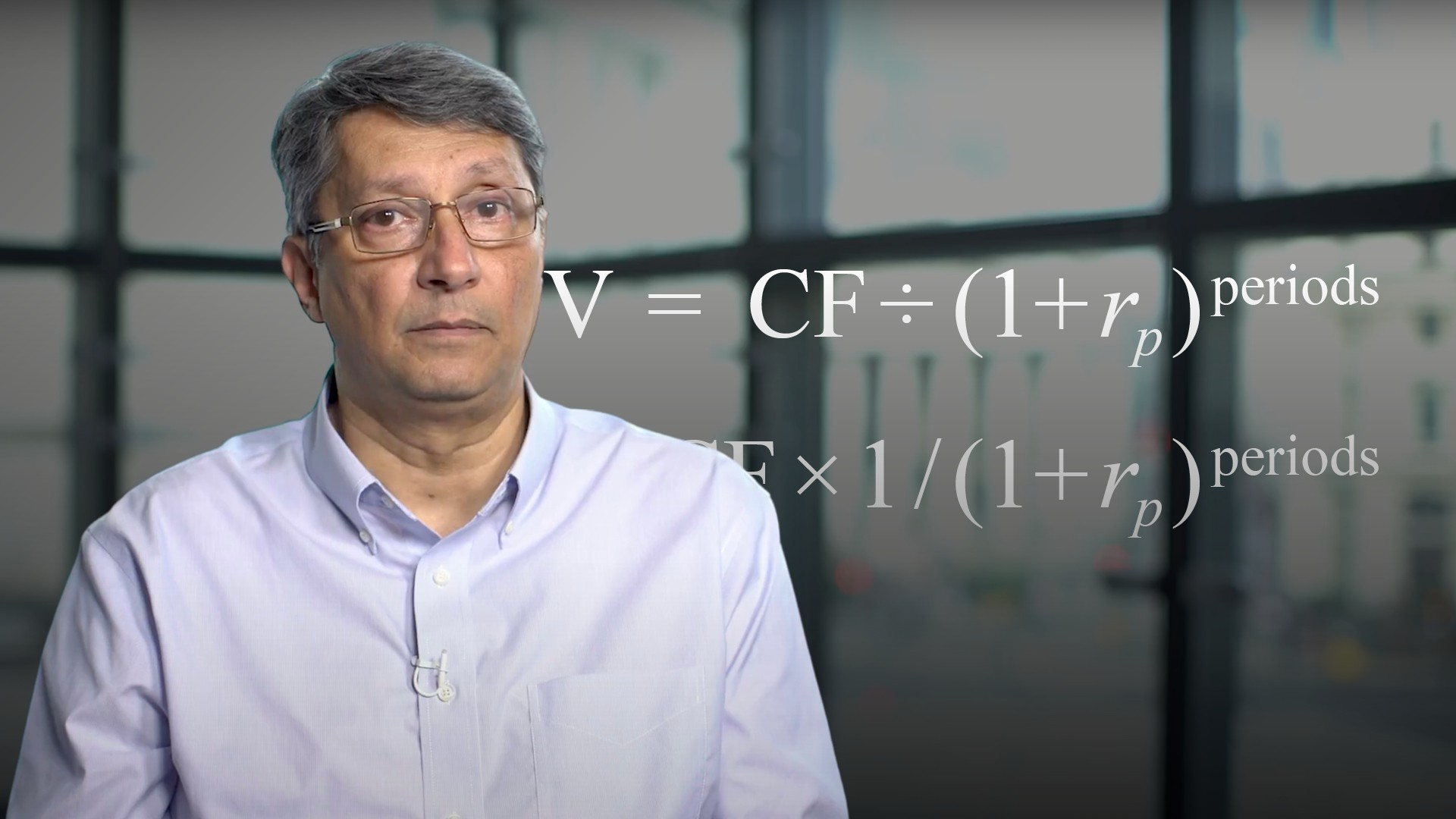04:19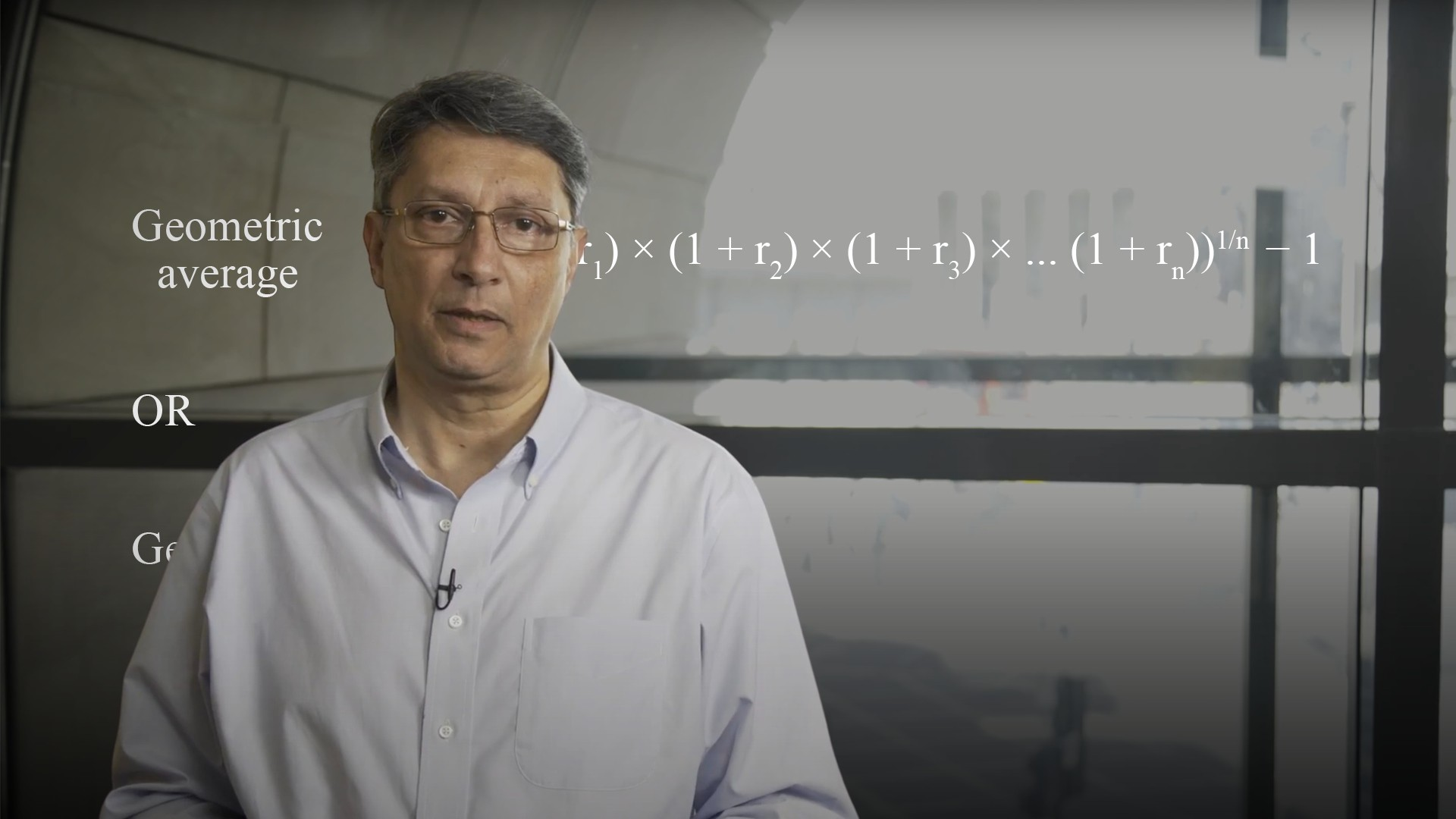04:4104:1605:2405:3906:13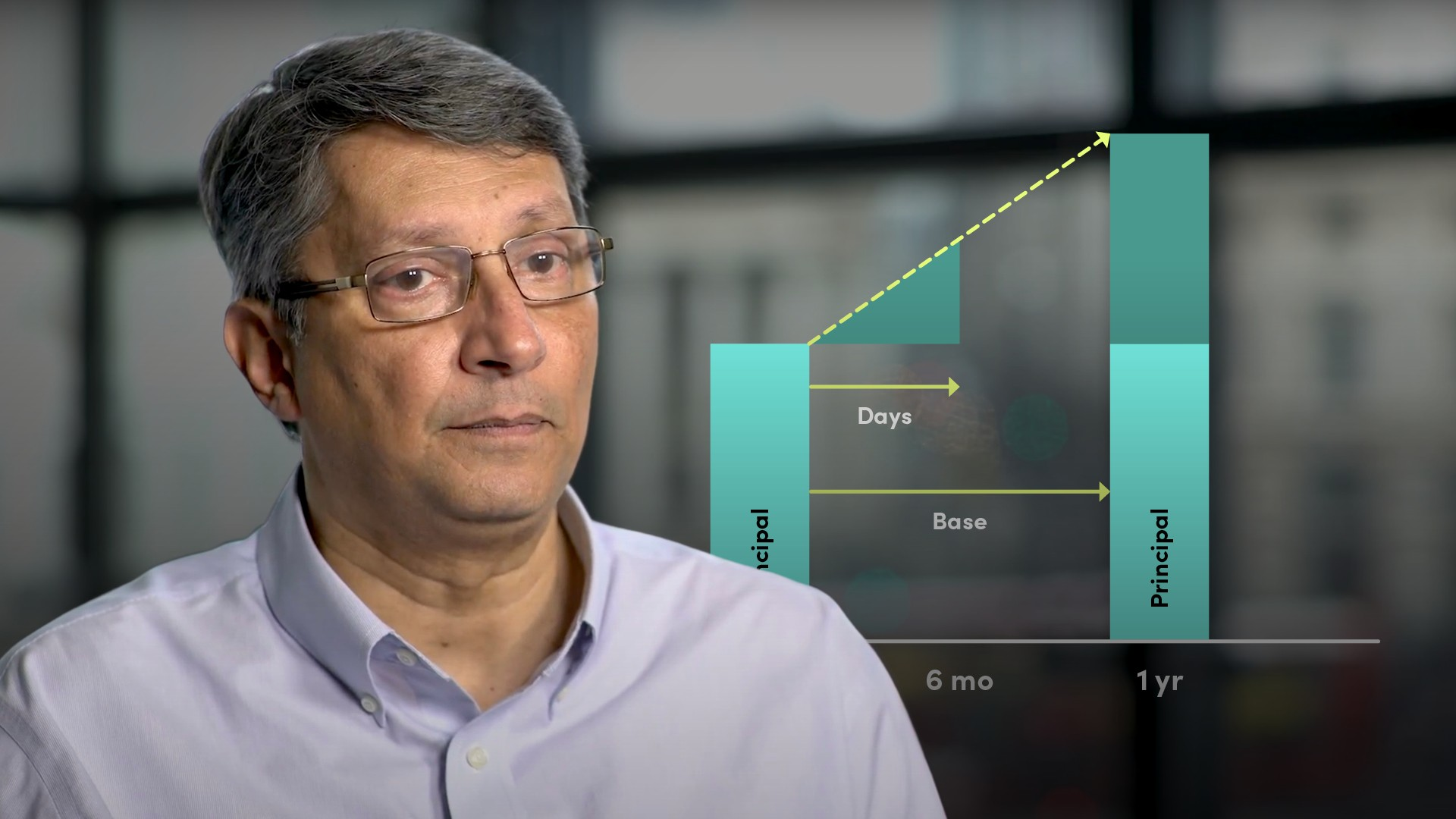05:2703:55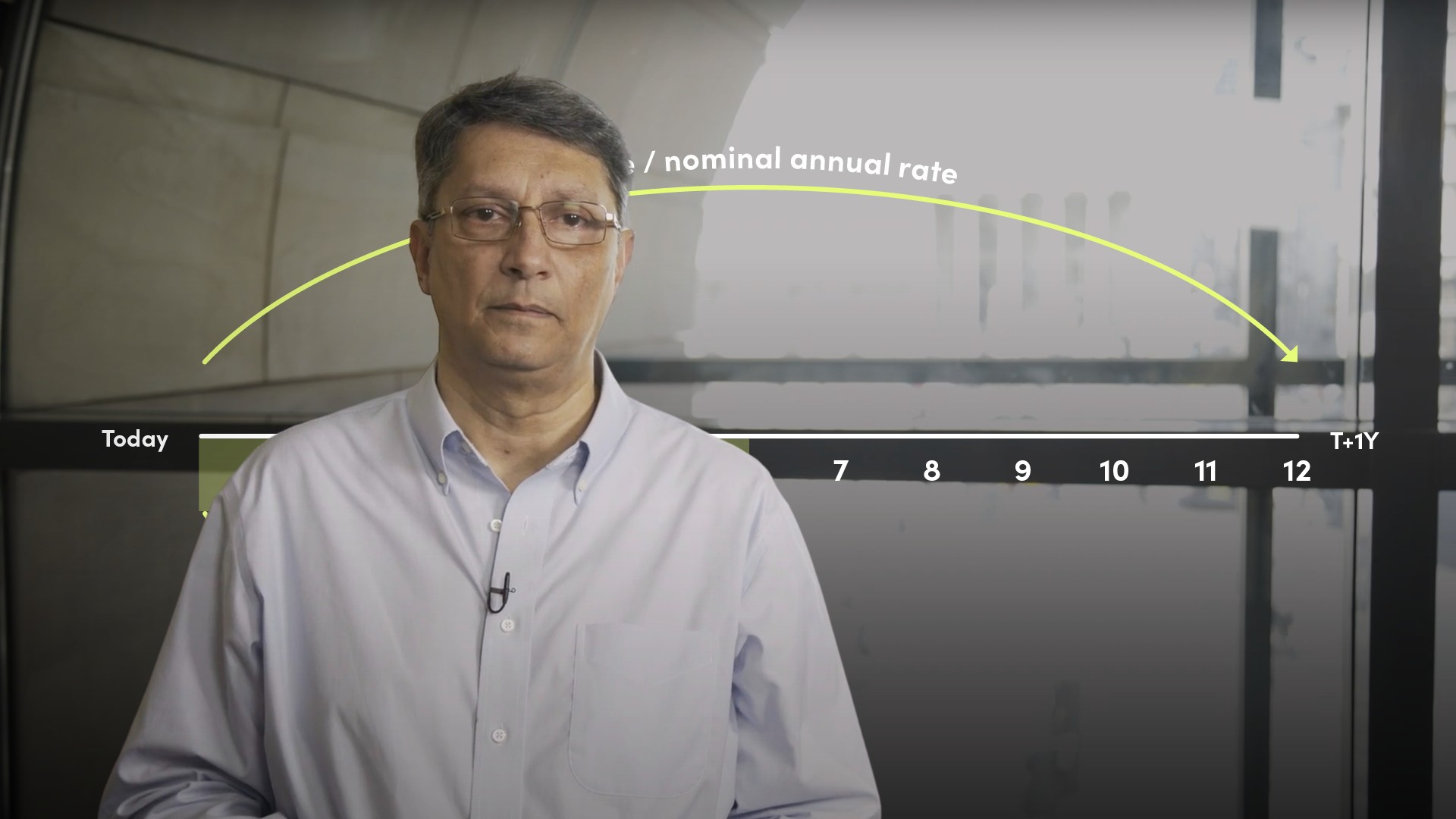07:58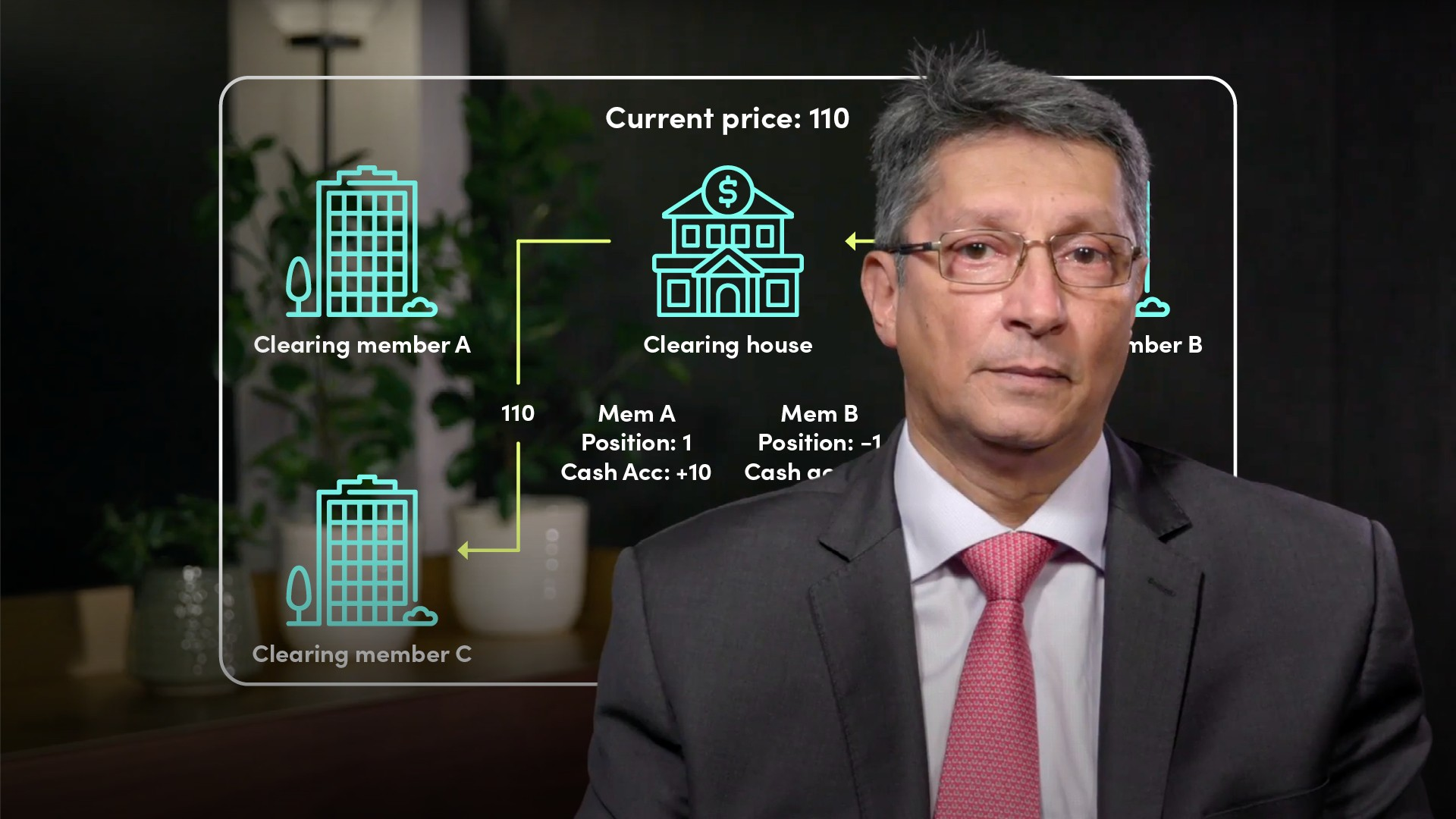05:2205:14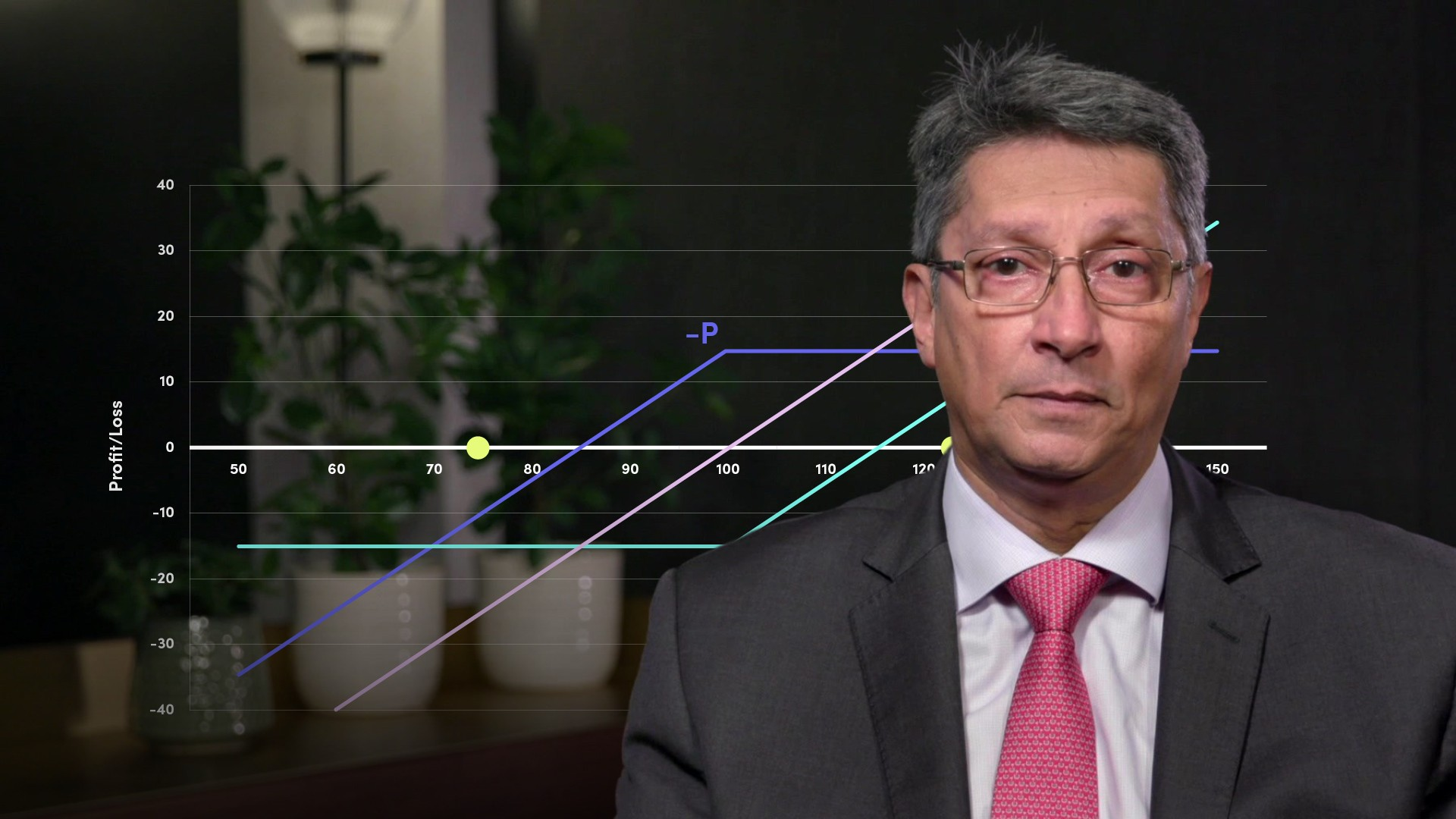06:0106:1306:23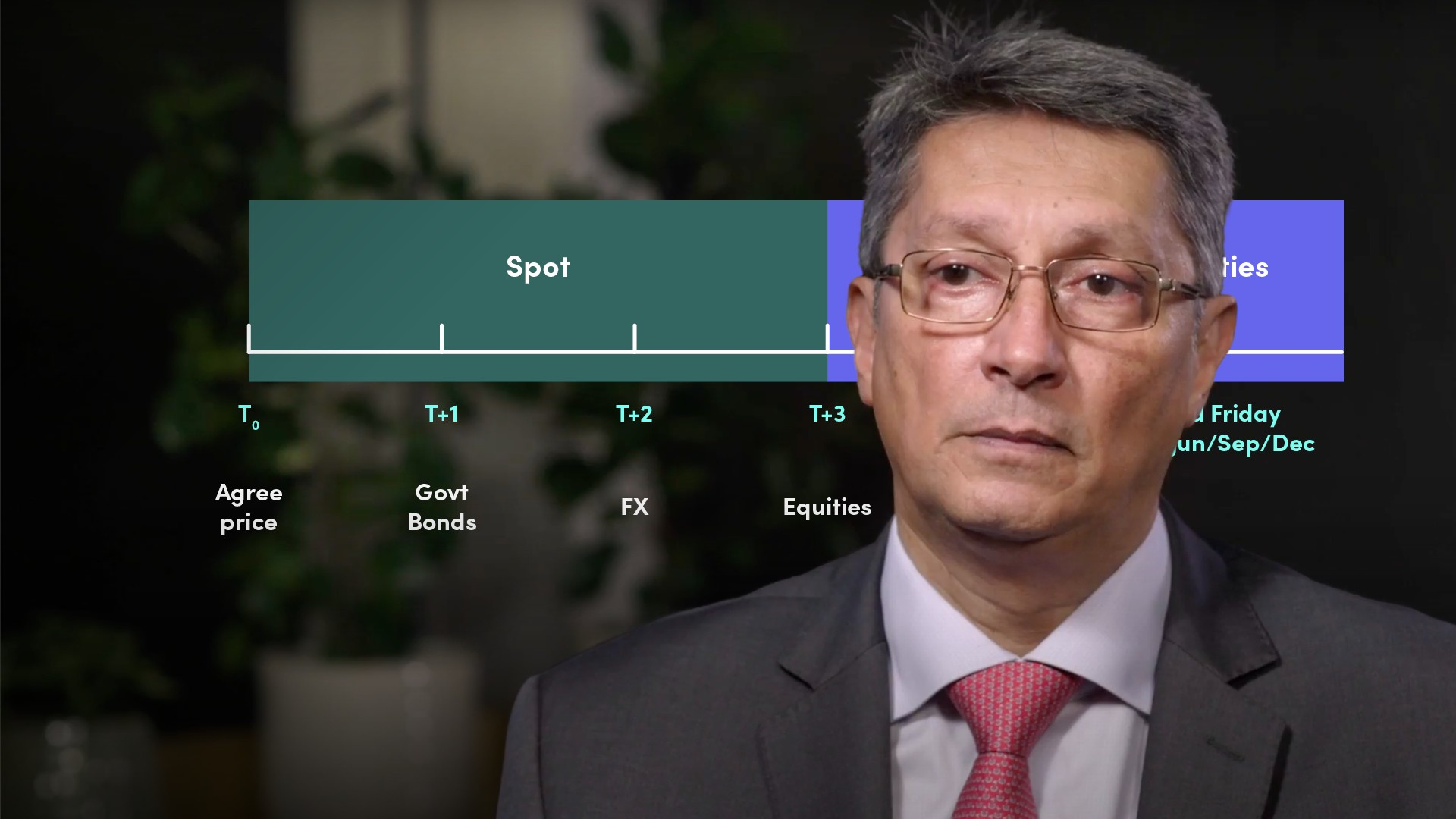05:3807:23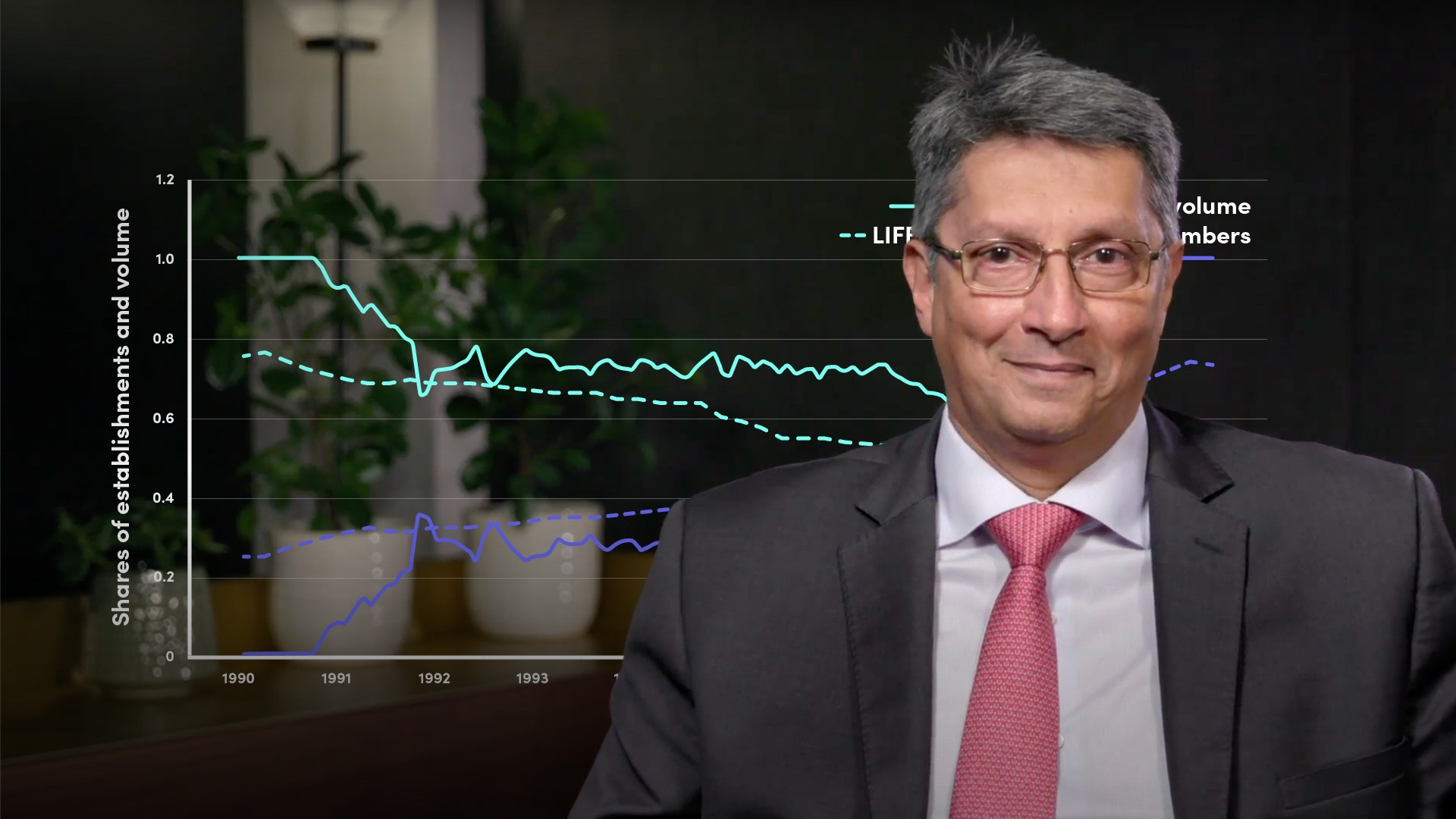04:01

## Related videosFinancial MathsFinancial MathsFinancial MathsFinancial MathsFinancial Maths### Accredited by